×
INTELLIGENT WORK FORUMS
FOR ENGINEERING PROFESSIONALS

Are you an
Engineering professional?
Join Eng-Tips Forums!
• Talk With Other Members
• Be Notified Of Responses
• Keyword Search
Favorite Forums
• Automated Signatures
• Best Of All, It's Free!

*Eng-Tips's functionality depends on members receiving e-mail. By joining you are opting in to receive e-mail.

#### Posting Guidelines

Promoting, selling, recruiting, coursework and thesis posting is forbidden.

# Force produced by pressurized water exiting a hose or pipe?4

## Force produced by pressurized water exiting a hose or pipe?

(OP)
Well known examples of the force produced by pressurized water exiting a pipe:

JetLev Water Propeled jet Pack.
https://youtu.be/n0109liIZGQ

Hovering Car.
https://youtu.be/s-2BN6FLDjA

I need to calculate the maximum for the thrust produced when the hose or pipe is bent rearward.

Since this is similar to the case of a water bottle rocket, the guess is it should be the same as that case:

The thrust force is:
F = 2*(P - P₀)*An,

Where P is the pressure in the pipe, P₀ is the ambient air pressure, and An is the area of the nozzle.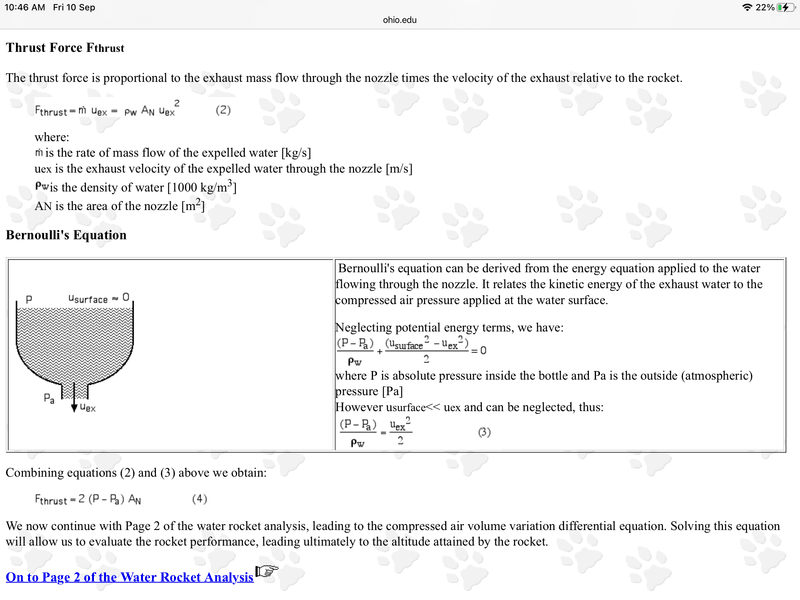https://www.ohio.edu/mechanical/thermo/property_ta...

Note the extra factor of 2 there. An odd aspect of this formula is that it would appear that you could just increase the thrust by making the nozzle size larger.

However, note in the derivation you need the cross-sectional area to be small enough on exit so that, by the Bernoulli principle, the exit velocity would be large. So making the exit area larger would obviate the argument used.

Here is the issue though. Another formula used for calculating the force required to hold a pipe in place because of the force produced on the bend due to the momentum of the water flow may, or may not, give a different answer.

See the calculations here: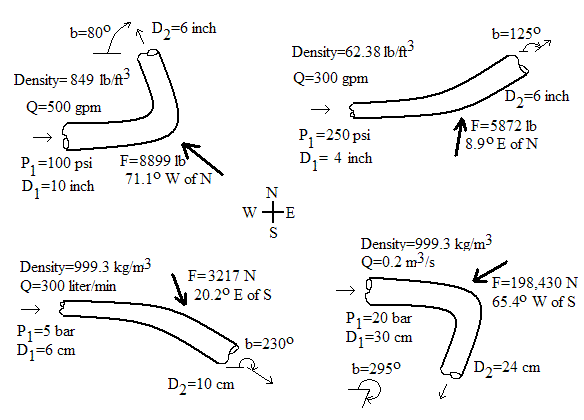Equations for force on pipe bend calculation
The equations used in our calculation can be found in nearly any college level fluid mechanics textbook (e.g. Munson et al., 1998) or fluid mechanics reference handbook. The force equations are based on linear momentum conservation. P₂ is computed using the Bernoulli Equation, which assumes negligible friction loss around the bend.
Subscript 1 is upstream of bend; Subscript 2 is downstream of bend; Subscript x is x-component of force; Subscript y is y-component of force. For a pipe bend in the horizontal plane where friction effects around the bend are negligible:
Fx = -P₁A₁- P₂A₂ cos(b) - d Q [V₁ + V₂ cos(b)]
Fy = P₂A₂ sin(b) + d V₂ Q sin(b) F = (Fx2 + Fy2)1/2
Q=VA, A=π D2 / 4
P₂ = P₁ + d (V₁2 - V₂2) / 2
Variables
To use the equations above, a consistent set of units must be used. The variables below show the SI (System International) units for each variable. Our calculation allows a variety of other units with the conversions made internally within the program.
A=Pipe flow area (m2)
b=Pipe angle shown in the figure above (valid range is 0 to 2π radians or 0 to 360o)
d=Liquid (or gas) mass density (kg/m3)
D=Pipe diameter (m)
F=Resistive (reaction) force (N)
P=Gauge pressure (N/m2, relative to atmospheric pressure

https://www.lmnoeng.com/Force/ForceBend.php

For the scenario we are considering, the hose is bent backwards, so the angle b in the formula is 0. So,

Fx = -P₁A₁ - P₂A₂ - d Q [V₁ + V₂]
Fy = 0

The minus sign is because the formula is calculating the force required to oppose the force on the pipe. Let’s look at the case, where the cross-sectional area is not changed. Then, it would appear, P₁ = P₂, and A₁ = A₂, V₁ = V₂. Then Fx = - 2PA - 2dQV.

For the scenario we are considering the pressure is high, but the speed V of the flow is low. Then the calculated force would be approx. Fx = -2PA, i.e., the force by the water on the pipe is Fx = 2PA.

The problem is this would be much larger than the water rocket formula since it uses the full cross-sectional area of the pipe, not just that of the nozzle.

Perhaps the problem is for the formula to be correctly applied what should be done is is for the exit pressure P₂ we should use the pressure of the ambient air, much smaller than the initial pressure in the pipe of P1. In that case the P₂A₂ term is approx. 0, so can be neglected. But what should we take for V₂?

By the Bernoulli formula for P₂ being greatly reduced that should ramp up the velocity V₂. Then V₂ would be much larger than V₁ and we can neglect dQV₁, so Fx = -P₁A₁ - dQV₂. We still have that force being much larger than the water bottle rocket case because A₁ is the area of the full hose/pipe not that of a nozzle.

So what is the real force being produced? This is something that can be tested for pressure washers. You would bend the hose backwards, and measure the force on the hose tending to force the hose away from you.

Pressure washers are provided with various nozzle sizes. The force produced should also be measured for all the nozzles provided as well as for the case with no nozzle.

Robert Clark

### RE: Force produced by pressurized water exiting a hose or pipe?

(OP)
Here is the water bottle rocket analysis for one pressure washer:We’ll use the first equation shown there which amounts to F = (mass flow rate)*(exit velocity). Take for example this pressure washer:

PowerShot PS4240 4200 PSI at 4.0 GPM Cold Water Gas Pressure Washer with HONDA GX390.
https://www.homedepot.com/p/SIMPSON...her-with-HON...

It can do 4,200 PSI at 4 GPM with a hose at 3/8 inch inner diameter. We’ll convert to metric to have consistent units. A gallon is 3.78 liters so 4 GPM, is 15.12 liters per minute which for water means 15.12 kg per minute or 0.25 kg/s.

For the exit speed, we’ll use the last formula given there,

(P - Pa)/ρw = uex2/2.

Now 4,200psi is 4,200/14.7 = 286 bar = 286E5 Pascals.

Then uex2 = 2*286E5/1000 = 57,000, and uex = 240 m/s.

So the force would be 0.25 * 240 = 60 N, or 6 kg-force, about 12 pounds.

On the other hand, let’s see what the answer would be using the online calculator:

Robert Clark

### RE: Force produced by pressurized water exiting a hose or pipe?

(OP)
...And here is the answer using the online calculator at the link:
https://www.lmnoeng.com/Force/ForceBend.php

We'll restrict the diameter at after the bend to make the scenario like the water bottle rocket case. I was surprised to see that the force was still much higher than the water bottle rocket case: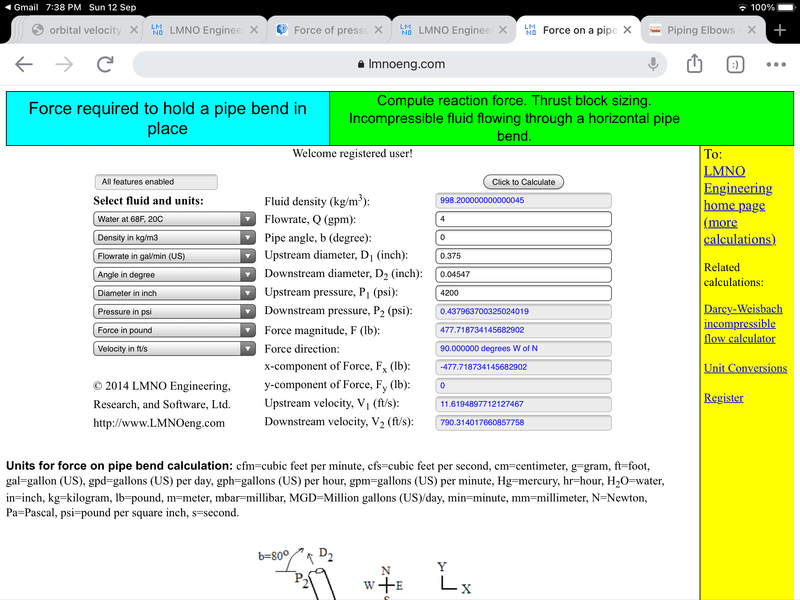The force calculates out to be 477 pounds! This compared to the ca. 12 pounds you get from the water bottle rocket analysis.

Note the exit gauge pressure is approx. 0, i.e., relative to that of ambient air, and the flow speed is greatly increased. Like in the water bottle rocket. Yet the force is still much higher. This now is a real discrepancy.

So which is the right answer?

Robert Clark

### RE: Force produced by pressurized water exiting a hose or pipe?

It looks to me like both sources are using the same equations, i.e.,

P2 = P1 + d (V1^2 - V2^2) / 2

and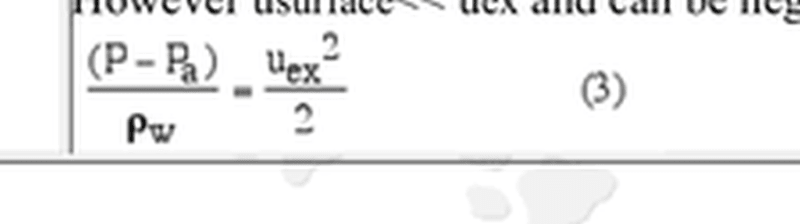So any discrepancy would be due to using different values

TTFN (ta ta for now)
I can do absolutely anything. I'm an expert! https://www.youtube.com/watch?v=BKorP55Aqvg
FAQ731-376: Eng-Tips.com Forum Policies forum1529: Translation Assistance for Engineers Entire Forum list http://www.eng-tips.com/forumlist.cfm

### RE: Force produced by pressurized water exiting a hose or pipe?

(OP)
The only obvious difference between the two cases is that the water bottle rocket case exits out to the open air, while in the force on a pipe bend case it remains inside the pipe. I thought I was taking that into account though in the pipe bend analysis by restricting the pipe diameter down just like a nozzle for the water bottle rocket. Note the pressure after the pipe bend does indeed equal ambient air pressure, like with the water bottle rocket exit flow, and the flow speed after the pipe bend also equals the exit speed for the water bottle rocket. (The online calculator for the pipe bend gives the speed in ft/s but it equals the speed for the water bottle rocket given in m/s.)

If the difference really is just that one exits out to the open air, then if we have a pressure washer and just put a bend in the hose with a restricted hose diameter then it would experience such a high force on the bend. That doesn’t seem reasonable since such pressure washers are in common use.

Well, perhaps the answer is that we are restricting the diameter in the pipe bend case and that is why the calculated force is so high. But actually if we don’t restrict the pipe or hose diameter then the force is even higher on the bend! See the attached calculation. If we keep the same pipe diameter after the bend the force on the bend is 928 pounds!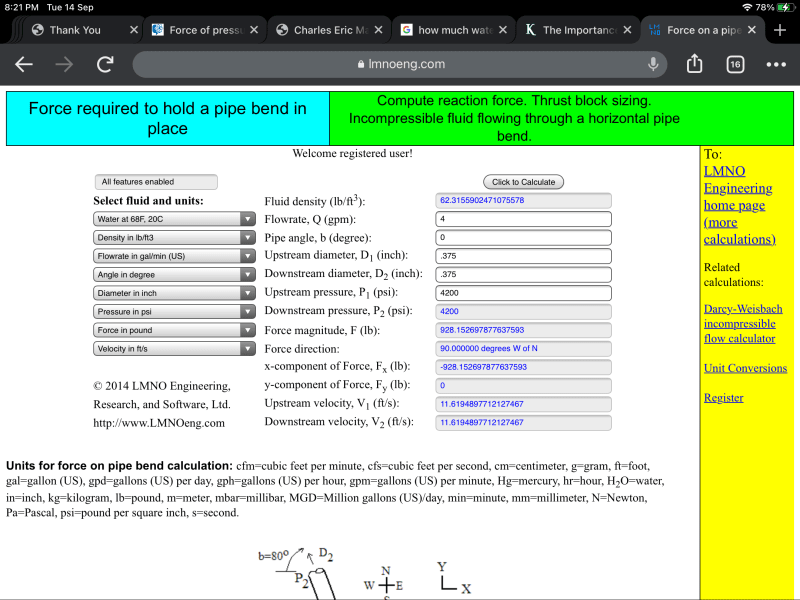Robert Clark

### RE: Force produced by pressurized water exiting a hose or pipe?

Surely with b = 0 you have a U bend?

This looks nothing like the rocket which is all in a straight line so the angle would be 180 degrees in your online form?

But is there a point to this?

Remember - More details = better answers
Also: If you get a response it's polite to respond to it.

### RE: Force produced by pressurized water exiting a hose or pipe?

#### Quote (LittleInch)

But is there a point to this?

Robert - I sure hope you're just looking at potential reaction forces and not trying to use this energy for lift still. Your drone is already going to be larger than most helicopters to lift this weight. A force at the nozzle or bend of somewhere in the neighborhood of 12-500lb is still going to be orders of magnitude less than your system weight. Not to mention the fact that you can't use a pump for lift during your entire flight.

### RE: Force produced by pressurized water exiting a hose or pipe?

Oh, it's the magic drone fighting bush fires thread....

Remember - More details = better answers
Also: If you get a response it's polite to respond to it.

### RE: Force produced by pressurized water exiting a hose or pipe?

(OP)
Littleinch, the point is to produce a lift force with a water jet, like in the videos in the first post. So you would need to know what is the max force possible. The hose has to be bent rearward to be able to make the lifting force.

The problem is which is the correct value for the thrust force produced. The value calculated by the water bottle rocket seems more likely. But on the other hand the value calculated by the force on the pipe bend is well established for actual applications yet it seems to give numbers that just seem unrealistic. A thousand pound force on a pipe bend on a pipe less than 1/2” wide??? Every time you put a bend in the hose of a pressure washer at 4,200 psi???

Note even just the mid level pressure washers at ca. 2,000 psi would still produce forces of hundreds of pounds according to the pipe bend formula.

Robert Clark

### RE: Force produced by pressurized water exiting a hose or pipe?

(OP)
Rputin, the pressurized water can certainly provide a lifting force even to carry a heavy payload, as shown in the videos in the first post. The question is how much lifting force?

By the way, pressure washers are in common use nowadays and anyone can do a simple test to find out which is the correct calculation.

Robert Clark

### RE: Force produced by pressurized water exiting a hose or pipe?

"anyone can do a simple test"

Yes, yes you can.

### RE: Force produced by pressurized water exiting a hose or pipe?

(OP)
3DDave, I intend to!

By the way look at one of the numerous videos of people using pressure washers on the net:

Pressure Washer Buying Guide | Consumer Reports.
https://youtu.be/6g3IYVsBc6k

Just from normal use you sometimes get bends in the hose. It doesn’t seem reasonable that this results in hundreds of pounds of force acting on those bends.

Robert Clark

### RE: Force produced by pressurized water exiting a hose or pipe?

Thanks. I've seen pressure washers. Just hoping you would go get actual experience to answer all the questions you have.

### RE: Force produced by pressurized water exiting a hose or pipe?

The pipeline thrust block analysis is not rocket science. The problem is the FBD, free body diagrams you have imagined are not the same for rocket's and pipeline bends. Well, not usually anyways. Along with setting the bend angle error that LittleInch mentioned, the 4200psi x area is an external force applied to a pipeline bend free body diagram, however that force would not be present on a free body diagram of a rocket, because the 4200psi remains wholely contained inside the rocket's tank. The rocket free body diagram would only use the downstream half of the pipe calculation, exit pressure x the area of the nozzle. There is no 4200psi x area of the 0.375" diameter pipe that would cause or resist the motion of the rocket. That's just an internal tank pressure.

In pipeline work, P x A are large forces and m/2g V^2 are usually very much smaller. For rocket work it is the exact opposite, P x A is very small and the m/2g V^2 is huge. Aerodynamic drag replaces the 4200psi x Area. In fact, many thrust block calculations you see on the Internet do not even take the change of momentum into consideration! They assume V is small enough to completely ignore. That's usually true enough, because they are not high velocity pressure washers. High velocities in pipelines cost too much money. Those calculations use only the P x (A1 sin Ø - Ao) to calculate forces on a bend.

A rocket Bernoulli equation would have 4200 psi acting down on the water surface, not 0, and there would also be a top of the tank, and sides, all having the same, counteracting 4200 psi pressure acting up. Net zero, up, down, left and right. No motion caused, no motion resisted. No P, only Pa x Ugx.

Rocket Science is here http://www.braeunig.us/space/propuls.htm

### RE: Force produced by pressurized water exiting a hose or pipe?

For your purposes forget the bend calculation.

What you're calculating there is really just end cap force.

Pressure washer tubing is actually really quite strong in an axial sense as it has to resist this force when operating and you release the trigger.

Jetting water out of a nozzle is something quite different.

Remember - More details = better answers
Also: If you get a response it's polite to respond to it.

### RE: Force produced by pressurized water exiting a hose or pipe?

The thickness of the material would have to be a factor. Explore K-factor involving the hydraulic calculations for Fire Sprinkler Systems. Hazen Williams co efficient.

#### Red Flag This Post

Please let us know here why this post is inappropriate. Reasons such as off-topic, duplicates, flames, illegal, vulgar, or students posting their homework.

#### Red Flag Submitted

Thank you for helping keep Eng-Tips Forums free from inappropriate posts.
The Eng-Tips staff will check this out and take appropriate action.

#### Resources

Low-Volume Rapid Injection Molding With 3D Printed Molds
Learn methods and guidelines for using stereolithography (SLA) 3D printed molds in the injection molding process to lower costs and lead time. Discover how this hybrid manufacturing process enables on-demand mold fabrication to quickly produce small batches of thermoplastic parts. Download Now
Examine how the principles of DfAM upend many of the long-standing rules around manufacturability - allowing engineers and designers to place a partâ€™s function at the center of their design considerations. Download Now
Industry Perspective: Education and Metal 3D Printing
Metal 3D printing has rapidly emerged as a key technology in modern design and manufacturing, so itâ€™s critical educational institutions include it in their curricula to avoid leaving students at a disadvantage as they enter the workforce. Download Now

Close Box

# Join Eng-Tips® Today!

Join your peers on the Internet's largest technical engineering professional community.
It's easy to join and it's free.

Here's Why Members Love Eng-Tips Forums:

•Talk To Other Members
• Notification Of Responses To Questions
• Favorite Forums One Click Access
• Keyword Search Of All Posts, And More...

Register now while it's still free!